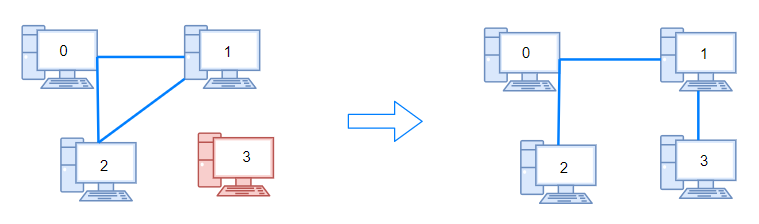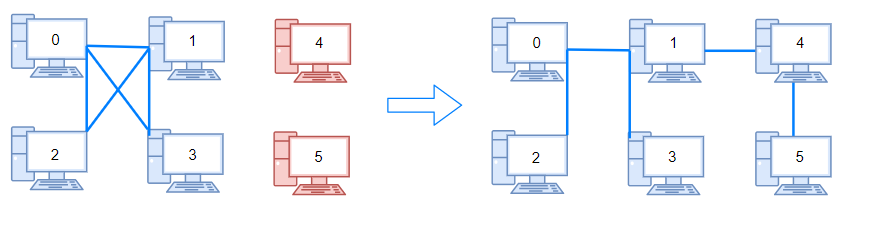0%

• 133 Clone Graph
• 207 Course Schedule
• 210 Course Schedule II
• 547 Number of Provinces
• 684 冗余连接
• 803 打砖块
• 947 移除最多的同行或同列石头
• 1202. Smallest String With Swaps
1. 连通网络的操作次数

### 133. leetcode Clone Graph

Clone an undirected graph. Each node in the graph contains a label and a list of its neighbors.

### 207. leetcode Course Schedule

There are a total of n courses you have to take, labeled from 0 to n - 1.

Some courses may have prerequisites, for example to take course 0 you have to first take course 1, which is expressed as a pair: [0,1]

Given the total number of courses and a list of prerequisite pairs, is it possible for you to finish all courses?

For example:

There are a total of 2 courses to take. To take course 1 you should have finished course 0. So it is possible.

There are a total of 2 courses to take. To take course 1 you should have finished course 0, and to take course 0 you should also have finished course 1. So it is impossible.

1. 每次找入度为0的点，输出该入度为0的点，并删除与之相连接的边
2. 重复1直到没有入度为0的点。之前输出入度为0的点若小于原图的结点数，那么说明图有环，即拓扑排序不存在，否则即为拓扑排序。

1. 在(a)图中v0和v1的入度都为0，选择v0并输出，接着删去顶点v0及出边<0,2>，得到的结果如(b)图所示。

2. 在(b)图中只有一个入度为0的顶点v1，输出v1，接着删去v1和它的三条出边<1,2>,<1,3>和<1,4>，得到的结果如(c)图所示。

3. 在(c)图中v2和v4的入度都为0，不妨选择v2并输出之，接着删去v2及两条出边<2,3>和<2,5>，得到的结果如(d)图所示。

4. 在(d)图上依次输出顶点v3,v4和v5，并在每个顶点输出后删除该顶点及出边。

C++

### 210. leetcode Course Schedule II

There are a total of n courses you have to take, labeled from 0 to n - 1.

Some courses may have prerequisites, for example to take course 0 you have to first take course 1, which is expressed as a pair: [0,1]

Given the total number of courses and a list of prerequisite pairs, return the ordering of courses you should take to finish all courses.

There may be multiple correct orders, you just need to return one of them. If it is impossible to finish all courses, return an empty array.

For example:

There are a total of 2 courses to take. To take course 1 you should have finished course 0. So the correct course order is [0,1]

There are a total of 4 courses to take. To take course 3 you should have finished both courses 1 and 2. Both courses 1 and 2 should be taken after you finished course 0. So one correct course order is [0,1,2,3]. Another correct ordering is[0,2,1,3].

### 547. 省份数量

n 个城市，其中一些彼此相连，另一些没有相连。如果城市 a 与城市 b 直接相连，且城市 b 与城市 c 直接相连，那么城市 a 与城市 c 间接相连。

• 1 <= n <= 200
• n == isConnected.length
• n == isConnected[i].length
• isConnected[i][j]10
• isConnected[i][i] == 1
• isConnected[i][j] == isConnected[j][i]

### 684. 冗余连接

• 输入的二维数组大小在 3 到 1000。
• 二维数组中的整数在1到N之间，其中N是输入数组的大小。

### 778. 水位上升的泳池中游泳

1. 2 <= N <= 50.
2. grid[i][j][0, ..., N*N - 1] 的排列。

### 803. 打砖块

• 一块砖直接连接到网格的顶部，或者
• 至少有一块相邻（4 个方向之一）砖块 稳定 不会掉落时

• m == grid.length
• n == grid[i].length
• 1 <= m, n <= 200
• grid[i][j]01
• 1 <= hits.length <= 4 * 104
• hits[i].length == 2
• 0 <= xi <= m - 1
• 0 <= yi <= n - 1
• 所有 (xi, yi) 互不相同

1. 消除所有hits的砖块，即将grid中在hits数组对应的位置上都设置为0
2. 可以首先将与屋顶连接的砖块看成同一个连通分量，因为和任意一个连接都没有什么区别。因此用并查集将他们合并
3. 初始化其他的砖块，将数值为1的合并起来
4. 经过2和3，就形成了若干个连通分量
5. 逆序遍历hits数组，对位置x ,y ：
1. 首先确保这个位置原先是1，否则相当于hit了空的位置，这里不该补上1
2. 如果x == 0说明这个砖块是和屋顶直接连接的，要先和屋顶合并一下（否则可能这个砖头连接的其他的砖头都不和屋顶连接了）
3. 合并x, y周围的联通分量
4. pre_num为合并前屋顶联通分量的儿子的数目，after_num为合并后的数量，则通过补上x,y 连接了after_num - pre_num - 1的数量

### 947. 移除最多的同行或同列石头

n 块石头放置在二维平面中的一些整数坐标点上。每个坐标点上最多只能有一块石头。

• 1 <= stones.length <= 1000
• 0 <= xi, yi <= 10^4
• 不会有两块石头放在同一个坐标点上

### 959. 由斜杠划分区域

（请注意，反斜杠字符是转义的，因此 \"\\" 表示。）。

1. 1 <= grid.length == grid.length <= 30
2. grid[i][j]'/''\'、或 ' '

• 空格：则合并4个区域
• /： 合并03, 12
• \: 合并 01, 23

### 1202. 交换字符串中的元素

• 1 <= s.length <= 10^5
• 0 <= pairs.length <= 10^5
• 0 <= pairs[i], pairs[i] < s.length
• s 中只含有小写英文字母

### 1319. 连通网络的操作次数• 1 <= n <= 10^5
• 1 <= connections.length <= min(n*(n-1)/2, 10^5)
• connections[i].length == 2
• 0 <= connections[i], connections[i] < n
• connections[i] != connections[i]
• 没有重复的连接。
• 两台计算机不会通过多条线缆连接。

### 1489. 找到最小生成树里的关键边和伪关键边

• 2 <= n <= 100
• 1 <= edges.length <= min(200, n * (n - 1) / 2)
• edges[i].length == 3
• 0 <= fromi < toi < n
• 1 <= weighti <= 1000
• 所有 (fromi, toi) 数对都是互不相同的。

• 初始化一个新的并查集，将除了mst_i外的所有边都合并
• 遍历edges，若能找不到边能生成MST或者两个连通分量的权值会增加，则说明是关键边，否则为伪关建边

### 1631. 最小体力消耗路径

• rows == heights.length
• columns == heights[i].length
• 1 <= rows, columns <= 100
• 1 <= heights[i][j] <= 106